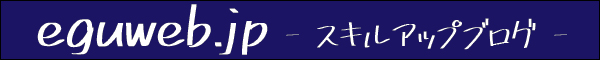# [DATEVALUE function] Convenient function to extract serial number from date/time data

You can use the DATEVALUE function to extract the serial number from date/time data.

## What is the DATEVALUE function?

A function that can extract the serial number from date/time data.

``DATEVALUE(date_string)``

Example of use:

``````=DATEVALUE("1969-7-20")

=DATEVALUE("1969/7/20")

=DATEVALUE(A2)

## Using the DATEVALUE function

For example, you can use it in the following situations.

1. I want to know the serial number from the date cell
2. I want to remove the time value because the time is included in the timestamp

Especially in the case of No. 2, there are cases where it can be used, so let’s try a concrete example.

Sets the cell value by cell reference.

Then the time part will be 0:00:00.

Let’s convert it to numeric notation.

All serial numbers are now integers!

## summary

With this kind of feeling, it returns and displays the serial value from the date (time).